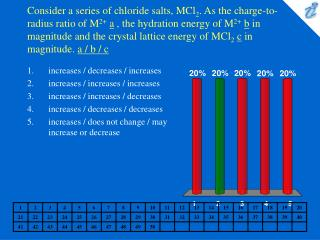DownloadDownload Presentationincreases / decreases / increases increases / increases / increases increases / increases / decreases increases / decrea

# increases / decreases / increases increases / increases / increases increases / increases / decreases increases / decrea

Télécharger la présentation## increases / decreases / increases increases / increases / increases increases / increases / decreases increases / decrea

- - - - - - - - - - - - - - - - - - - - - - - - - - - E N D - - - - - - - - - - - - - - - - - - - - - - - - - - -
##### Presentation Transcript

1. Consider a series of chloride salts, MCl2. As the charge-to-radius ratio of M2+a , the hydration energy of M2+b in magnitude and the crystal lattice energy of MCl2c in magnitude. a / b / c • increases / decreases / increases • increases / increases / increases • increases / increases / decreases • increases / decreases / decreases • increases / does not change / may increase or decrease

2. Which of the following metal ions has the most negative heat of hydration? • Ca2+ • K+ • Mn2+ • Li + • Al3+

3. To calculate the molality of a solution, which of the following must be measured? I. mass of solute II. mass of solvent III. total volume of solution • I and II • I and III • II and III • only I • I, II and III

4. Calculate the molality of a solution prepared by dissolving 150. grams of sodium dichromate dihydrate, Na2Cr2O7 · 2H2O in 350. gm of water. • 1.44 m • 1.56 m • 1.79 m • 1.86 m • 2.30 m

5. Calculate the mole fraction of C2H5OH in a solution that contains 46 grams of ethyl alcohol, C2H5OH, and 64 grams of methyl alcohol, CH3OH. • 0.33 • 0.42 • 0.50 • 0.67 • 1.5

6. At 25°C a solution consists of 0.450 mole of pentane, C5H12, and 0.250 mole of cyclopentane, C5H10. What is the mole fraction of pentane in the vapor that is in equilibrium with this solution? The vapor pressure of the pure liquids at 25°C are 451 torr for pentane and 321 torr for cyclopentane. Assume that the solution is an ideal solution. • 0.284 • 0.551 • 0.716 • 0.643 • 0.357

7. The freezing point of a solution made by dissolving 0.200 g of a molecular compound in 100. g of H2O is - 0.0120°C. Calculate the molecular weight of the compound. The compound does not ionize in water. Kf for H2O = 1.86°C/m. • 155 amu • 215 amu • 310 amu • 395 amu • 450 amu

8. When 27.0 g of an unknown nonelectrolyte is dissolved in 250.0 mL of benzene, the solution boils at 82.5°C. Calculate the molecular weight of the unknown nonelectrolyte. The Kb for benzene = 2.53°C/m. The boiling point of pure benzene is 80.1°C. The density of benzene = 0.876 g/mL. • 60 g/mol • 130 g/mol • 220 g/mol • 110 g/mol • 150 g/mol

9. A 0.0100 m solution of HX, a weak acid, freezes at - 0.02000°C. Calculate the % ionization of HX. Kf for water = 1.86°C/m. • 2.3% • 7.5% • 9.5% • 11% • 18%

10. K2SO4 dissolves in water according to K2SO4 {image} 2K+ + SO42- A 0.100 m solution of K2SO4 freezes at - 0.432&#176;C. Calculate the apparent percent dissociation of K2SO4in this solution. Kffor water = 1.86&#176;C/m. • 62% • 64% • 66% • 68% • 83%

11. Which of the following is not an advantage of osmotic pressure over boiling point elevation and freezing point depression for the determination of molecular weights? • The relatively large change in osmotic pressure is easier to measure accurately than the small boiling point elevation. • The relatively large change in osmotic pressure can be determined without the special apparatus needed for accurate measurement of the smaller freezing point depression. • A more dilute solution can be used for osmotic pressure measurements thereby saving money when the molecular weights of expensive substances are to be determined. • Use of a more dilute solution allows determinations for sparingly soluble high- molecular-weight substances. • Osmotic pressure can be used to accurately determine the molecular weight of impure substances.